# Faculty & Staff

## Faculty

•Research interests - computational complexity theory and design of algorithms, and their applications in bioinformatics, biomolecular computation, hardware verification, and combinatorial auctions.

•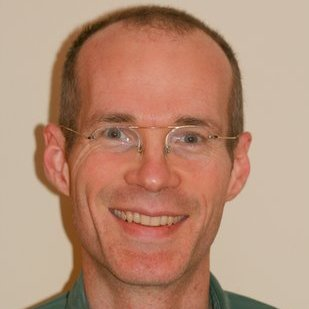Research interests - computational geometry, graph drawing, information theory, data compression, and algorithms in general.

•Research interests - Laplacians, eigenvalues, and expansion, primarily on graphs. Also explicit construction questions, primarily from theoretical computer science.

•Research interests - convex optimization, numerical methods.

•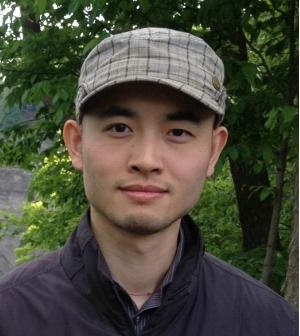Research interests - algorithmic game theory, auctions, pricing.

•Research interests - algorithm design, combinatorial optimization, application of algorithms in computer systems and machine learning.

•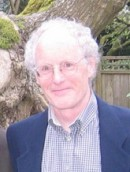Research interests - computational complexity, graph theory and computational geometry.

•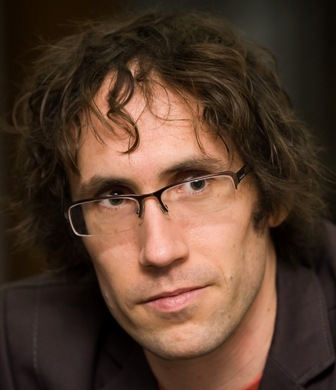Research interests - algorithm design, combinatorial optimization, application of algorithms in computer systems and machine learning.

•Research interests - machine learning, convex optimization.

•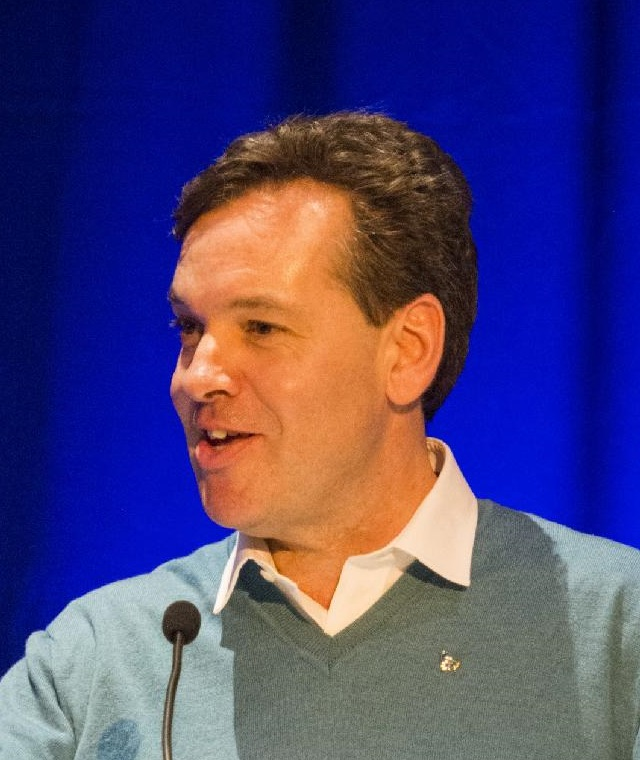Research interests - combinatorial optimization, network design, approximation algorithms, graph theory.

## Affiliated Faculty

•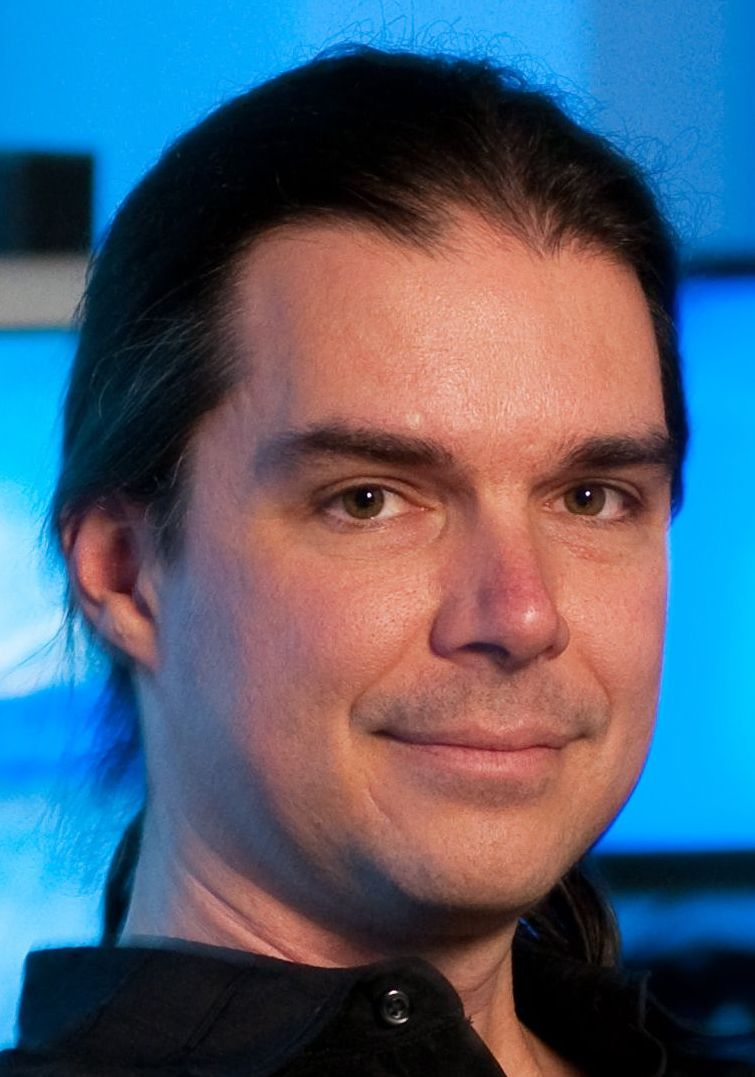Research interests - algorithmics, bioinformatics, computational intelligence and computer music.

•Research interests - network flows, discrete optimization.

•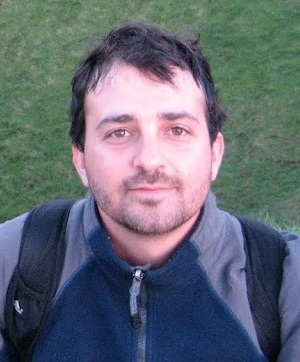Research interests - high-dimensional probability, compressed sensing.

•Research interests - submodular optimization, discrete optimization.

## Staff

Holly Kwan, Group Assistant
hmitchel@cs.ubc.ca | 604-822-3060